# Traveling Big Gun Sprinkler

Use this form to calculate the water application depth for a traveling big gun sprinkler based on the sprinkler flow rate, travel speed, and travel lane spacing. Learn more about the units used on this page.Sprinkler Flow Rate:
Irrigation Efficiency:
%
Travel Lanes Width (distance between sets):
Travel Speed:
Net Water Application Depth:

## The Equation

This calculator uses this formula to determine the Net Water Application Rate of a traveling big gun sprinkler.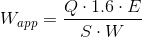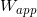= Net Water Application (in)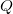= Flow Rate from the sprinkler(gpm)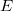= Irrigation Efficiency (as a decimal, or %/100)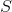= Travel Speed of the Big Gun Sprinkler (ft/min)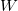= Travel lanes width (ft)

WSU Prosser - IAREC, 24106 N Bunn Rd, Prosser WA 99350-8694, 509-786-2226. Contact Us